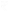# Bank of India EMI Calculator for loans

BOI Calculator helps you to calculate monthly EMI with graphs, Total amount with Interest, Total interest amount & yearly interest amount with loan calculator of Bank of India.

Calculate Loans EMI Online

Loan Amount
Interest Rate (Reducing) % Per Annum
Loan Tenure (in Months)

Monthly EMITotal Amount with InterestTotal Interest AmountYearly Interest Amount